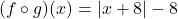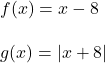Question

f(x) = x − 8, g(x) = |x + 8|
Find
(f ∘ g)(x).

1.##Step-by-step explanation:To find (f ∘ g)(x) , substitute g(x) into f(x). That is for every x in f (x) replace it with g (x)

That’s

###Hope this helps you